# Tricking Neural Networks: Create your own Adversarial Examples

10 Jan 2018 | Daniel Geng and Rishi Veerapaneni

Assassination by neural network. Sound crazy? Well, it might happen someday, and not in the way you may think. Of course, neural networks could be trained to pilot drones or operate other weapons of mass destruction, but even an innocuous (and presently available) network trained to drive a car could be turned to act against its owner. This is because neural networks are extremely susceptible to something called adversarial examples.

Adversarial examples are inputs to a neural network that result in an incorrect output from the network. It’s probably best to show an example. You can start with an image of a panda on the left which some network thinks with 57.7% confidence is a “panda.” The panda category is also the category with the highest confidence out of all the categories, so the network concludes that the object in the image is a panda. But then by adding a very small amount of carefully constructed noise you can get an image that looks exactly the same to a human, but that the network thinks with 99.3% confidence is a “gibbon.” Pretty crazy stuff!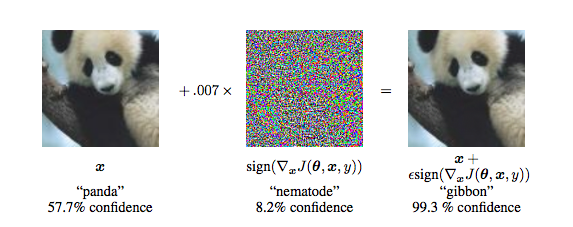From Explaining and Harnessing Adversarial Examples by Goodfellow et al.

So just how would assassination by adversarial example work? Imagine replacing a stop sign with an adversarial example of it–that is, a sign that a human would recognize instantly but a neural network would not even register. Now imagine placing that adversarial stop sign at a busy intersection. As self-driving cars approach the intersection the on-board neural networks would fail to see the stop sign and continue right into oncoming traffic, bringing its occupants to near certain death (in theory).

Now, this might just be one convoluted and (more than) slightly sensationalized instance of how people could use adversarial examples for harm, but there are many more. For example, the iPhone X’s “Face ID” unlocking feature relies on neural nets to recognize faces and is therefore susceptible to adversarial attacks. People could construct adversarial images to bypass the Face ID security features. Other biometric security systems would also be at risk and illegal or improper content could potentially bypass neural-network-based content filters by using adversarial examples. The existence of these adversarial examples means that systems that incorporate deep learning models actually have a very high-security risk.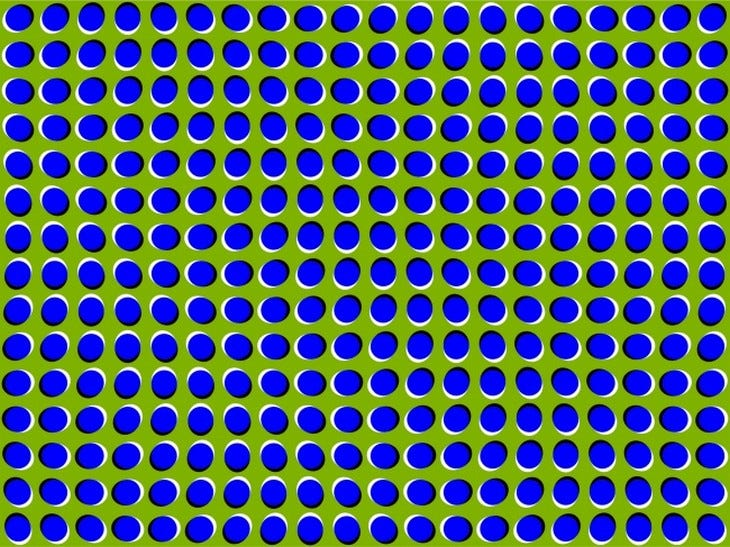You can understand adversarial examples by thinking of them as optical illusions for neural networks. In the same way, optical illusions can trick the human brain, adversarial examples can trick neural networks.

The above adversarial example with the panda is a targeted example. A small amount of carefully constructed noise was added to an image that caused a neural network to misclassify the image, despite the image looking exactly the same to a human. There are also non-targeted examples which simply try to find any input that tricks the neural network. This input will probably look like white noise to a human, but because we aren’t constrained to find an input that resembles something to a human the problem is a lot easier.

We can find adversarial examples for just about any neural network out there, even state-of-the-art models that have so-called “superhuman” abilities, which is slightly troubling. In fact, it is so easy to create adversarial examples that we will show you how to do it in this post. All the code and dependencies you need to start generating your own adversarial examples can be found in this GitHub repo.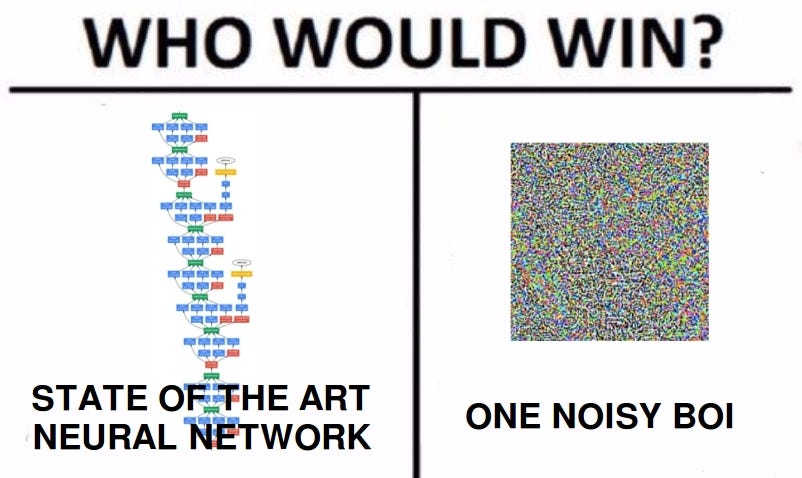A meme, extolling the effectivness of adversarial examples

### Adversarial Examples on MNIST

The code for this part can be found in this GitHub repo (but downloading the code isn’t necessary to understand this post):

We will be trying to trick a vanilla feedforward neural network that was trained on the MNIST dataset. MNIST is a dataset of 28×28 pixel images of handwritten digits. They look something like this: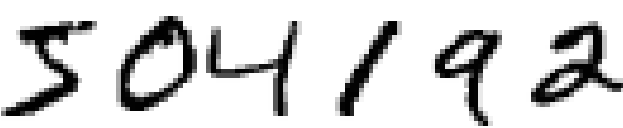6 MNIST images side-by-side

Before we do anything we should first import the libraries we’ll need.

``import network.network as networkimport network.mnist_loader as mnist_loaderimport pickleimport matplotlib.pyplot as pltimport numpy as np``

There are 50000 training images and 10000 test images. We first load up the pre-trained neural network (which is shamelessly stolen from this amazing introduction to neural networks):

``with open('trained_network.pkl', 'rb') as f:      net = pickle.load(f)      training_data, validation_data, test_data = mnist_loader.load_data_wrapper()``

For those of you unfamiliar with pickle, it’s a way for python to serialize data (i.e. write to disk) in essence saving classes and objects. Using `pickle.load()` just opens up the saved version of the network.

So a bit about this trained neural network. It has 784 input neurons (one for each of the 28×28 = 784 pixels), one layer of 30 hidden neurons, and 10 output neurons (one for each digit). All its activations are sigmoidal; its output is a one-hot vector indicating the network’s prediction, and it was trained by minimizing the mean squared error loss.

To show that the neural network is actually trained we can write a quick little function:

``def predict(n):    # Get the data from the test set    x = test_data[n]``
``    # Get output of network and prediction    activations = net.feedforward(x)    prediction = np.argmax(activations)``
``    # Print the prediction of the network    print('Network output: ')    print(activations)``
``    print('Network prediction: ')    print(prediction)``
``    print('Actual image: ')        # Draw the image    plt.imshow(x.reshape((28,28)), cmap='Greys')``

This method chooses the nth sample from the test set, displays it, and then runs it through the neural network using the `net.feedforward(x)` method. Here’s the output of a few images: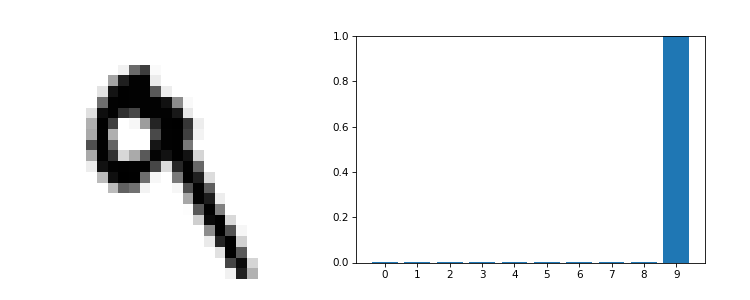The left side is the MNIST image. The right side plots the 10 outputs of the neural network, called activations. The larger the activation at an output the more the neural network thinks the image is that number.

Alright, so we have a trained network, but how are we going to trick it? We’ll first start with a simple non-targeted approach and then once we get that down we’ll be able to use a cool trick to modify the approach to work as a targeted approach.

### Non-Targeted Attack

The idea is to generate some image that is designed to make the neural network have a certain output. For instance, say our goal label/output is:

That is, we want to come up with an image such that the neural network’s output is the above vector. In other words, find an image such that the neural network thinks the image is a 5 (remember, we’re zero indexing). It turns out we can formulate this as an optimization problem in much the same way we train a network. Let’s call the image we want to make x⃗ (a 784 dimensional vector, because we flatten out the 28 × 28 pixel image to make calculations easier). We’ll define a cost function as:

Where the y_goal is our goal label from above. The output of the neural network given our image is y(x⃗). You can see that if the output of the network given our generated image x⃗ is very close to our goal label y_goal, then the corresponding cost is low. If the output of the network is very far from our goal then the cost is high. Therefore, finding a vector x⃗ that minimizes the cost CC results in an image that the neural network predicts as our goal label. Our problem now is to find this vector x⃗.

Notice that this problem is incredibly similar to how we train a neural network, where we define a cost function and then choose weights and biases (a.k.a. parameters) that minimize the cost function. In the case of adversarial example generation, instead of choosing weights and biases that minimize the cost, we hold the weights and biases constant (in essence hold the entire network constant) and choose an x⃗ input that minimizes the cost.

To do this, we’ll take the exact same approach used in training a neural network. That is, we’ll use gradient descent! We can find the derivatives of the cost function with respect to the input, ∇_xC, using backpropagation, and then use the gradient descent update to find the best x⃗ that minimizes the cost.

Backpropagation is usually used to find the gradients of the weights and biases with respect to the cost, but in full generality backpropagation is just an algorithm that efficiently calculates gradients on a computational graph (which is what a neural network is). Thus it can also be used to calculate the gradients of the cost function with respect to the inputs of the neural network.

Alright, let’s look at the code that actually generates adversarial examples:

``def adversarial(net, n, steps, eta):    """    net : network object        neural network instance to use    n : integer        our goal label (just an int, the function transforms it into a one-hot vector)    steps : integer        number of steps for gradient descent    eta : integer        step size for gradient descent    """    # Set the goal output    goal = np.zeros((10, 1))    goal[n] = 1``
``    # Create a random image to initialize gradient descent with    x = np.random.normal(.5, .3, (784, 1))``
``    # Gradient descent on the input    for i in range(steps):        # Calculate the derivative        d = input_derivative(net,x,goal)                # The GD update on x        x -= eta * d``
``    return x``

First, we create our y_goal, called `goal` in the code. Next we initialize our x⃗ as a random 784-dimensional vector. With this vector we can now start gradient descent, which is really only two lines of code. The first line `d = input_derivative(net,x,goal)` calculates ∇_xC using backpropagation (the full code for this is in the notebook for the curious, but we’ll skip describing it here as it’s really just a ton of math. If you want a very good description of what backprop is (which is what `input_derivative` is really doing) check out this website (incidentally, the same place we got the neural network implementation from)). The second and final line of the gradient descent loop, `x -= eta * d` is the update. We move in the direction opposite the gradient with step size `eta`.

Here are non-targeted adversarial examples for each class along with the neural network’s predictions: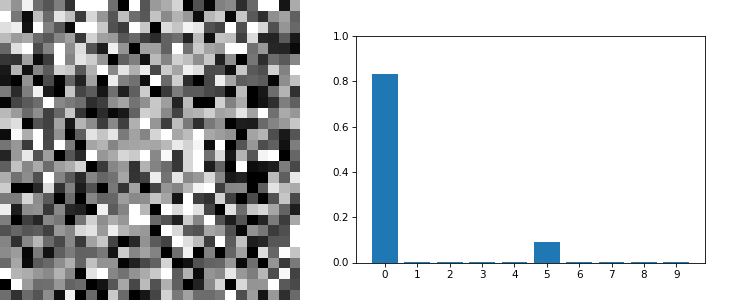The left side is the non-targeted adversarial exampele (a 28 X 28 pixel image). The right side plots the activations of the network when given the image.

Incredibly the neural network thinks that some of the images are actually numbers with a very high confidence. The “3” and “5” are pretty good examples of this. For most of the other numbers the neural network just has very low activations for every number indicating that it is very confused. Looks pretty good!

There might be something bugging you at this point. If we want to make an adversarial example corresponding to a five then we want to find a x⃗ that when fed into the neural network gives an output as close as possible to the one-hot vector representing “5”. However, why doesn’t gradient descent just find an image of a “5”? After all, the neural network would almost certainly believe that an image of a “5” was actually a “5” (because it is actually a “5”). A possible theory as to why this happens is the following:

The space of all possible 28×28 images is utterly massive. There are 256^(28×28)≈10^1888 possible different 28×28 pixel black and white images. For comparison, a common estimate for the number of atoms in the observable universe is 10^80. If each atom in the universe contained another universe then we would have 10^160 atoms. If each atom contained another universe whose atoms contained another universe and so on for about 23 times, then we would almost have reached 10^1888 atoms. Basically, the number of possible images is mind-bogglingly huge.

And out of all these photos only an essentially insignificant fraction actually look like numbers to the human eye. Whereas given that there are so many images, a good amount of them would look like numbers to a neural network (part of the problem is that our neural network was never trained on images that don’t look like numbers, so given an image that doesn’t look like a number the neural network’s outputs are pretty much random). So when we set off to find something that looks like a number to a neural network we’re much more likely to find an image that looks like noise or static than to find an image that actually looks like a number to a human just by sheer probability.

### Targeted Attack

These adversarial examples are cool and all, but to humans they just look like noise. Wouldn’t it be cool if we could have adversarial examples that actually looked like something? Maybe an image of a ‘2’ that a neural network thought was a 5? It turns out that’s possible! And moreover, with just a very small modification to our original code. What we can do is add a term to the cost function that we’re minimizing. Our new cost function will be:

Where xtargetxtarget is what we want our adversarial example to look like (x_target is therefore a 784 dimensional vector, the same dimension as our input). So what we’re doing now is we’re simultaneously minimizing two terms. The left term we’ve seen already. Minimizing this will make the neural network output ygoalygoal when given x⃗. Minimizing the second term will try to force our adversarial image x to be as close as possible to x_target as possible (because the norm is smaller when the two vectors are closer), which is what we want! The extra λ out front is a hyperparameter that dictates which of the terms is more important. As with most hyperparameters we find after a lot of trial and error that .05 is a good number to set λ to.

If you know about ridge regularization you might find the cost function above very very familiar. In fact, we can interpret the above cost function as placing a prior on our model for our adversarial examples.

If you don’t know anything about regularization, feel free to click here to find out more:

The code to implement minimizing the new cost function is almost identical to the original code (we called the function `sneaky_adversarial()` because we’re being sneaky by using a targeted attack. Naming is always the hardest part of programming…)

``def sneaky_adversarial(net, n, x_target, steps, eta, lam=.05):    """    net : network object        neural network instance to use    n : integer        our goal label (just an int, the function transforms it into a one-hot vector)    x_target : numpy vector        our goal image for the adversarial example    steps : integer        number of steps for gradient descent    eta : integer        step size for gradient descent    lam : float        lambda, our regularization parameter. Default is .05    """        # Set the goal output    goal = np.zeros((10, 1))    goal[n] = 1``
``    # Create a random image to initialize gradient descent with    x = np.random.normal(.5, .3, (784, 1))``
``    # Gradient descent on the input    for i in range(steps):        # Calculate the derivative        d = input_derivative(net,x,goal)                # The GD update on x, with an added penalty         # to the cost function        # ONLY CHANGE IS RIGHT HERE!!!        x -= eta * (d + lam * (x - x_target))``
``    return x``

The only thing we’ve changed is the gradient descent update: `x -= eta * (d + lam * (x - x_target))`. The extra term accounts for the new term in our cost function. Let’s take a look at the result of this new generation method: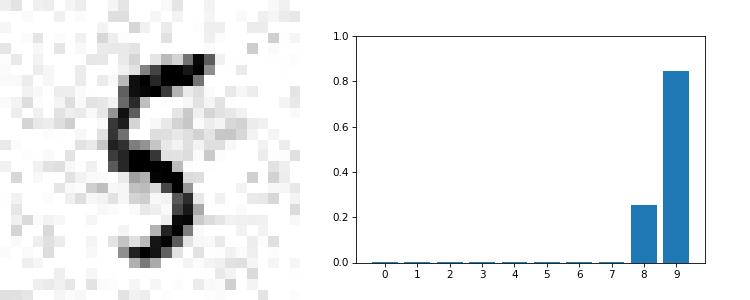The left side is the targeted adversarial example (a 28 X 28 pixel image). The right side plots the activations of the network when given the image.

Notice that as with the non-targeted attack there are two behaviors. Either the neural network is completely tricked and the activation for the number we want is very high (for example the “targeted 5” image) or the network is just confused and all the activations are low (for example the “targeted 7” image). What’s interesting though is that many more images are in the former category now, completely tricking the neural network as opposed to just confusing it. It seems that making adversarial examples that have been regularized to be more “number-like” tends to make convergence better during gradient descent.

### Protecting Against Adversarial Attacks

Awesome! We’ve just created images that trick neural networks. The next question we could ask is whether or not we could protect against these kinds of attacks. If you look closely at the original images and the adversarial examples you’ll see that the adversarial examples have some sort of grey tinged background.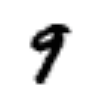Original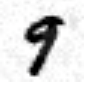Targeted

One naive thing we could try is to use binary thresholding to completely white out the background:

``def binary_thresholding(n, m):    """    n: int 0-9, the target number to match    m: index of example image to use (from the test set)    """        # Generate adversarial example    x = sneaky_generate(n, m)``
``# Binarize image    x = (x > .5).astype(float)        print("With binary thresholding: ")        plt.imshow(x.reshape(28,28), cmap="Greys")    plt.show()``
``# Get binarized output and prediction    binary_activations = net.feedforward(x)    binary_prediction = np.argmax(net.feedforward(x))        print("Prediction with binary thresholding: ")    print(binary_prediction)        print("Network output: ")    print(binary_activations)``

Here’s the result: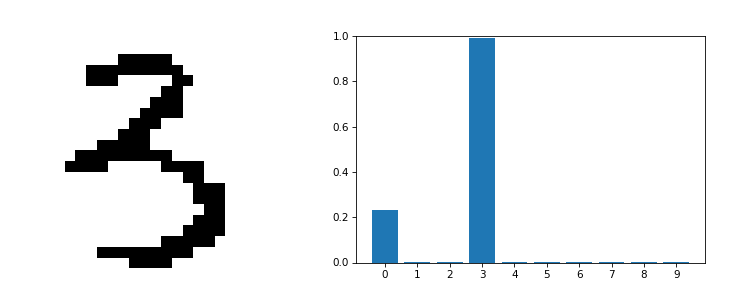The effect of binary thresholding on an MNIST adversarial image. The left image is the adversarial image, the right side is the binarized image

Turns out binary thresholding works! But this way of protecting against adversarial attacks is not very good. Not all images will always have an all white background. For example look at the image of the panda at the very beginning of this post. Doing binary thresholding on that image might remove the noise, but not without disturbing the image of the panda a ton. Probably to the point where the network (and humans) can’t even tell it’s a panda.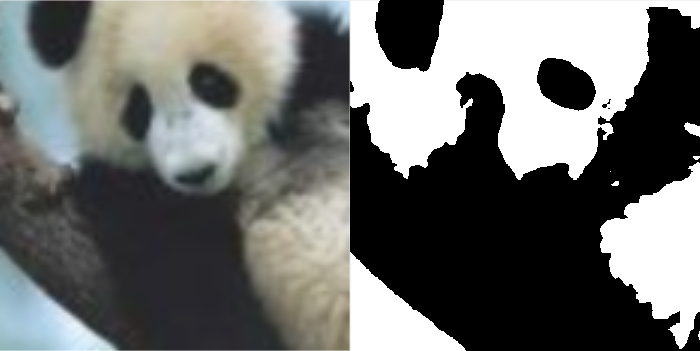Doing binary thresholding on the panda results in a blobby image

Another more general thing we could try to do is to train a new neural network on correctly labeled adversarial examples as well as the original training test set. The code to do this is in the ipython notebook (be aware it takes around 15 minutes to run). Doing this gives an accuracy of about 94% on a test set of all adversarial images which is pretty good. However, this method has it’s own limitations. Primarily in real life you are very unlikely to know how your attacker is generating adversarial examples.

There are many other ways to protect against adversarial attacks that we won’t wade into in this introductory post, but the question is still an open research topic and if you’re interested there are many great papers on the subject.

### Black Box Attacks

An interesting and important observation of adversarial examples is that they generally are not model or architecture specific. Adversarial examples generated for one neural network architecture will transfer very well to another architecture. In other words, if you wanted to trick a model you could create your own model and adversarial examples based off of it. Then these same adversarial examples will most probably trick the other model as well.

This has huge implications as it means that it is possible to create adversarial examples for a completely black box model where we have no prior knowledge of the internal mechanics. In fact, a team at Berkeley managed to launch a succesful attack on a commercial AI classification system using this method.

### Conclusion

As we move toward a future that incorporates more and more neural networks and deep learning algorithms in our daily lives we have to be careful to remember that these models can be fooled very easily. Despite the fact that neural networks are to some extent biologically inspired and have near (or super) human capabilities in a wide variety of tasks, adversarial examples teach us that their method of operation is nothing like how real biological creatures work. As we’ve seen neural networks can fail quite easily and catastrophically, in ways that are completely alien to us humans.

We do not completely understand neural networks and to use our human intuition to describe neural networks would be unwise. For example, often times you will hear people say something to the effect of “the neural network thinks the image is of a cat because of the orange fur texture.” The thing is a neural network does not “think” in the sense that humans “think.” They are fundamentally just a series of matrix multiplications with some added non-linearities. And as adversarial examples show us, the outputs of these models are incredibly fragile. We must be careful not to attribute human qualities to neural networks despite the fact that they have human capabilities. That is, we must not anthropomorphize machine learning models.A neural network trained to detect dumbbells “believes” that “dumbbells” are sometimes paired with a disembodied arm. Clearly not what we would expect. From Google Research.

All in all, adversarial examples should humble us. They show us that although we have made great leaps and bounds there is still much that we do not know.